09 子序列问题：详解重要的一大类动态规划问题

## 什么是子序列问题？

1. 题目涉及子序列；
2. 问题符合动归典型特征，特别是求“最”优解问题（最大值和最小值）；
3. 题目的答案是题设数组的子序列，或者来源于子序列。

## 最长回文子序列

示例1：


示例2：



### 算法问题分析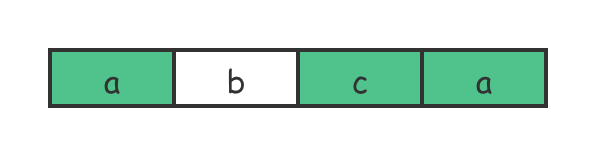1. 重叠子问题：在穷举的过程中肯定存在重复计算的问题。这是因为各种排列组合间肯定存在重叠子问题的情况；
2. 无后效性：对不是最长的回文子序列来说，它一定被包含在更长的回文子序列中。而更长的回文子序列不会包含在较短的回文子序列中，依赖是单向的；
3. 最优子结构：对整个字符串，其最长的回文子序列肯定包含了更短长度字符串中的回文子序列，因此子问题可以递归求解。

### 写出状态转移方程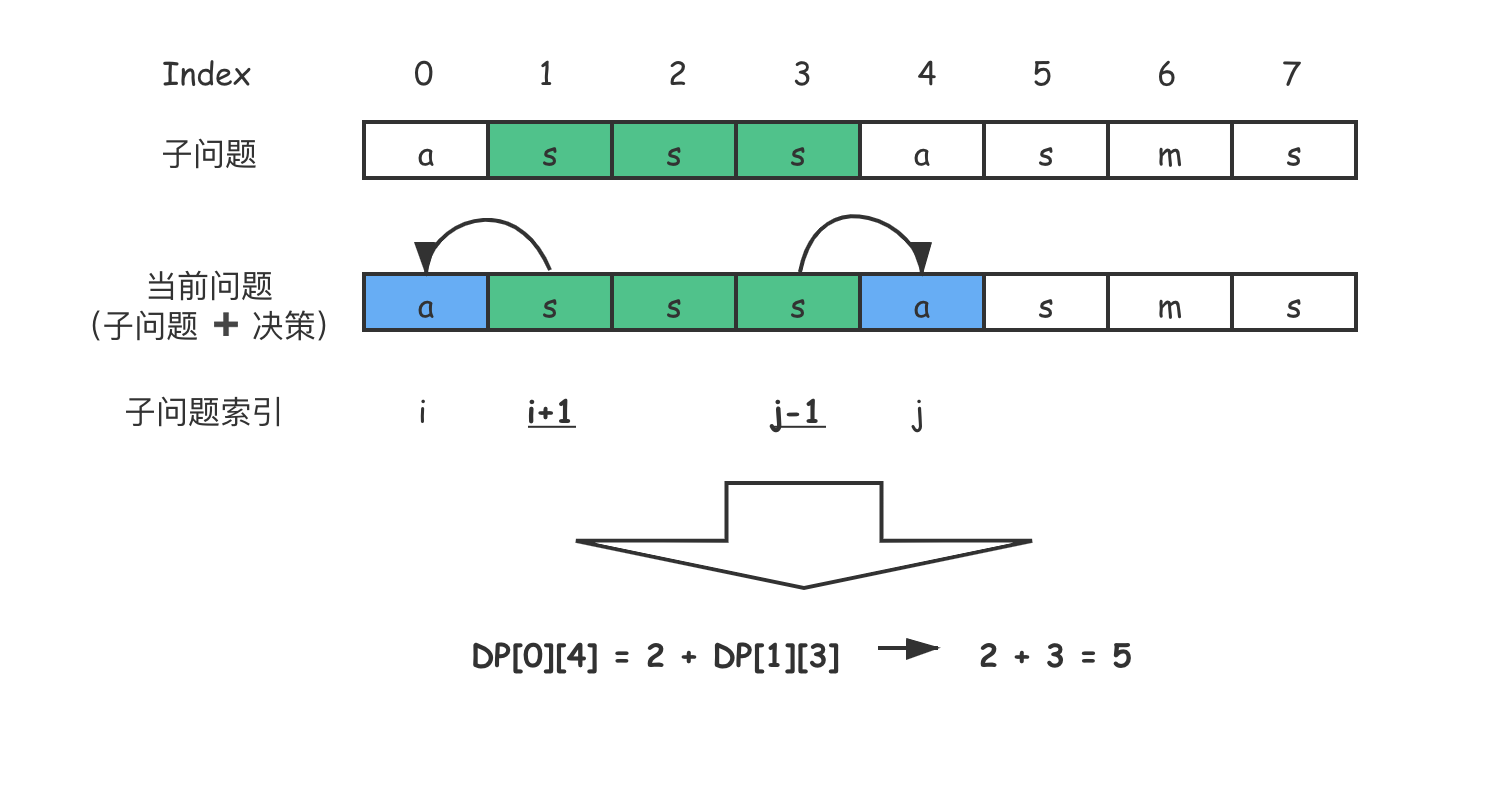1. 如果 $$s$i$ == s$j$$$（示意图是相等的），那么 $$DP$i$$j$ = 2 + DP$i+1$$j-1$$$
2. 如果 $$s$i$ != s$j$$$，就意味着 $$s$i$$$$$s$j$$$ 是不可能同时出现在 $$s$i … j$$$ 的最⻓回文子序列中的。这时我们该怎么做？这里需要进一步作出决策。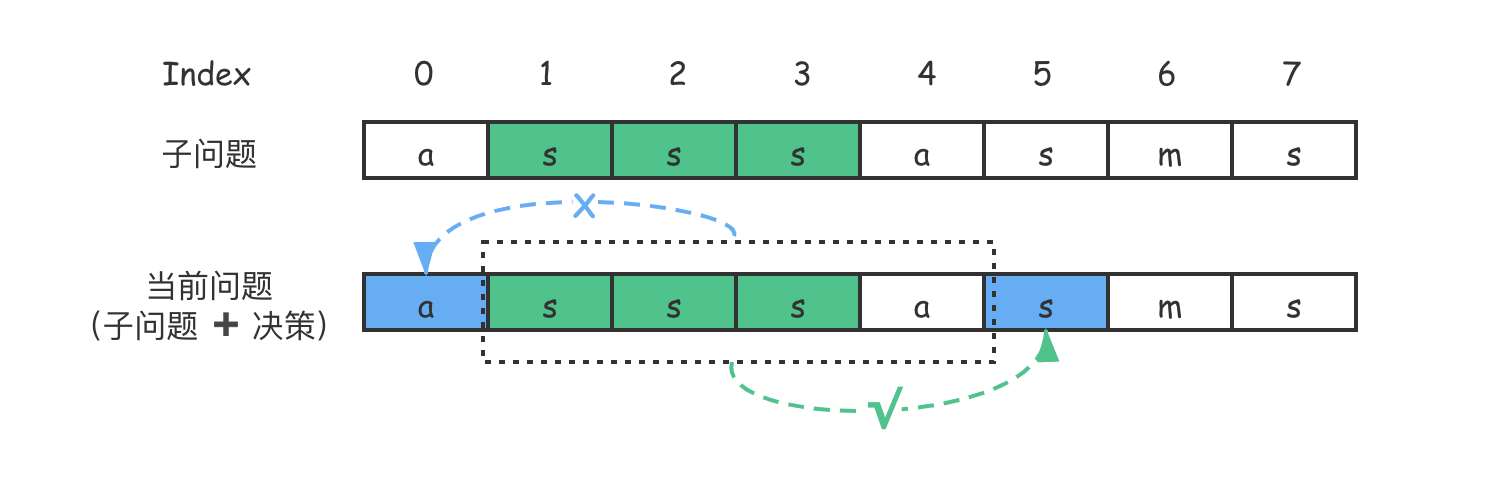$DP(i, j)=\\left\\{\\begin{array}{c}- 2 + DP\[i+1$$j-1$,\\ s$i$==s$j$\\\\\\- max(DP$i+1$$j$, DP$i$$j-1$),\\ s$i$ \\ne s$j$- \\end{array}\\right.\]

### 编写代码进行求解

Java 实现：

int getLongestPalindromeSubseq(String s) {
int n = s.length(); if (0 == n) return 0;

int[][] dp = new int[n][n];
for (int[] row : dp) { Arrays.fill(row, 0); }
for (int i = 0; i < n; i++) dp[i][i] = 1; // 初始化状态

for (int i = n-1; i >= 0; i--) {
for (int j = i+1; j < n; j++) {
if (s.charAt(i)==s.charAt(j)) {
dp[i][j] = 2 + dp[i+1][j-1];
} else {
dp[i][j] = Math.max(dp[i+1][j], dp[i][j-1]); // 作出进一步决策
}
}
}

return dp[n-1]; // 输出答案
}


C++ 实现：

int GetLongestPalindromeSubseq(string s) {
int n = static_cast<int>(s.size());
if (0 == n) return 0;

int dp[n][n]; memset(dp, 0, sizeof(dp));
for (int i = 0; i < n; i++) dp[i][i] = 1; // 初始化状态

for (int i = n-1; i >= 0; i--) {
for (int j = i+1; j < n; j++) {
if (s[i]==s[j]) {
dp[i][j] = 2 + dp[i+1][j-1];
} else {
dp[i][j] = max(dp[i+1][j], dp[i][j-1]); // 作出进一步决策
}
}
}

return dp[n-1]; // 输出答案
}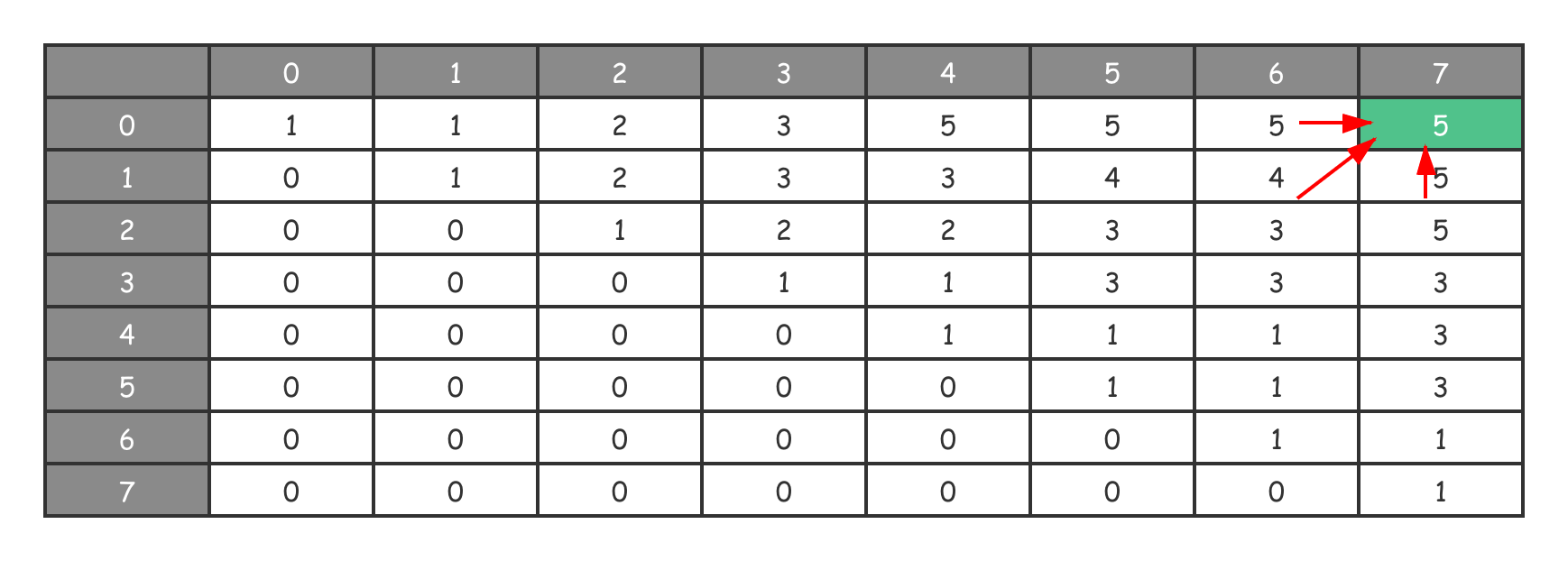## 最长公共子序列

• 1 ≤ text1.length ≤ 1000；
• 1 ≤ text2.length ≤ 1000；
• 输入的字符串只含有小写英文字符。
示例1：


示例2:



### 写出状态转移方程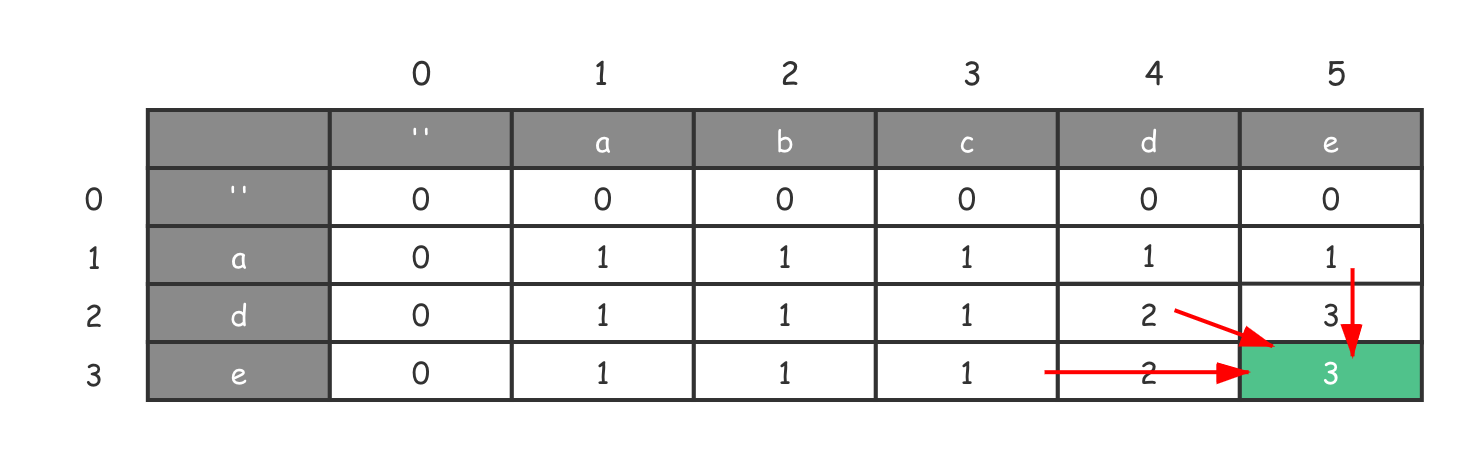1. $$text1$i-1$ == text2$j-1$$$，即当前遍历的两个字符在最长公共子序列中，此时 $$DP$i$$j$ = 1 + DP$i-1$$j-1$$$
2. $$text1$i-1$ != text2$j-1$$$，即当前遍历的两个字符至少有一个不在最长公共子序列中。仿照最长回文子序列的处理方法，由于两个字符至少有一个不在，因此我们需要丢弃一个。因此在不等的情况下，需要进一步作出决策。

$DP(i, j)=\\left\\{\\begin{array}{c}- dp\[i$$j$ = 1 + dp$i-1$$j-1$,\\ text1$i$==text2$j$\\\\\\- max(dp$i-1$$j$, dp$i$$j-1$),\\ text1$i$ \\ne text2$j$- \\end{array}\\right.\]

### 编写代码进行求解

Java 实现：

int getLongestCommonSubsequence(String text1, String text2) {
int m = text1.length(), n = text2.length();
int[][] dp = new int[m + 1][n + 1];
for (int[] row: dp) { Arrays.fill(row, 0); }

for (int j = 1; j <= n; j++) {
for (int i = 1; i <= m; i++) {
if (text2.charAt(j - 1) == text1.charAt(i - 1)) {
dp[i][j] = dp[i - 1][j - 1] + 1;
} else {
dp[i][j] = Math.max(dp[i - 1][j], dp[i][j - 1]);
}
}
}

return dp[m][n];
}


C++ 实现：

int GetLongestCommonSubsequence(string text1, string text2) {
int m = text1.size(), n = text2.size();
int dp[m+1][n+1]; memset(dp, 0, sizeof(dp)); // 多一行一列为了 i, j=1 时有 base value

for (int j = 1; j <= n; j++) {
for (int i = 1; i <= m; i++) {
if (text2[j-1]==text1[i-1]) {
dp[i][j] = dp[i-1][j-1] + 1;
} else {
dp[i][j] = max(dp[i-1][j], dp[i][j-1]);
}
}
}

return dp[m][n];
}


1. 如果当前字符相同，那么说明当前遍历的两个字符均在最长公共子序列中，因此需要将当前序列长度加 1。由于之前的序列长度在 $$dp$i-1$$j-1$$$，因此结果是 $$dp$i-1$$j-1$ + 1$$
2. 如果当前字符不同，我们需要从之前的序列中选取一个最大的，也就是从 $$dp$i-1$$j$$$$$dp$i$$j-1$$$ 中取最大值。